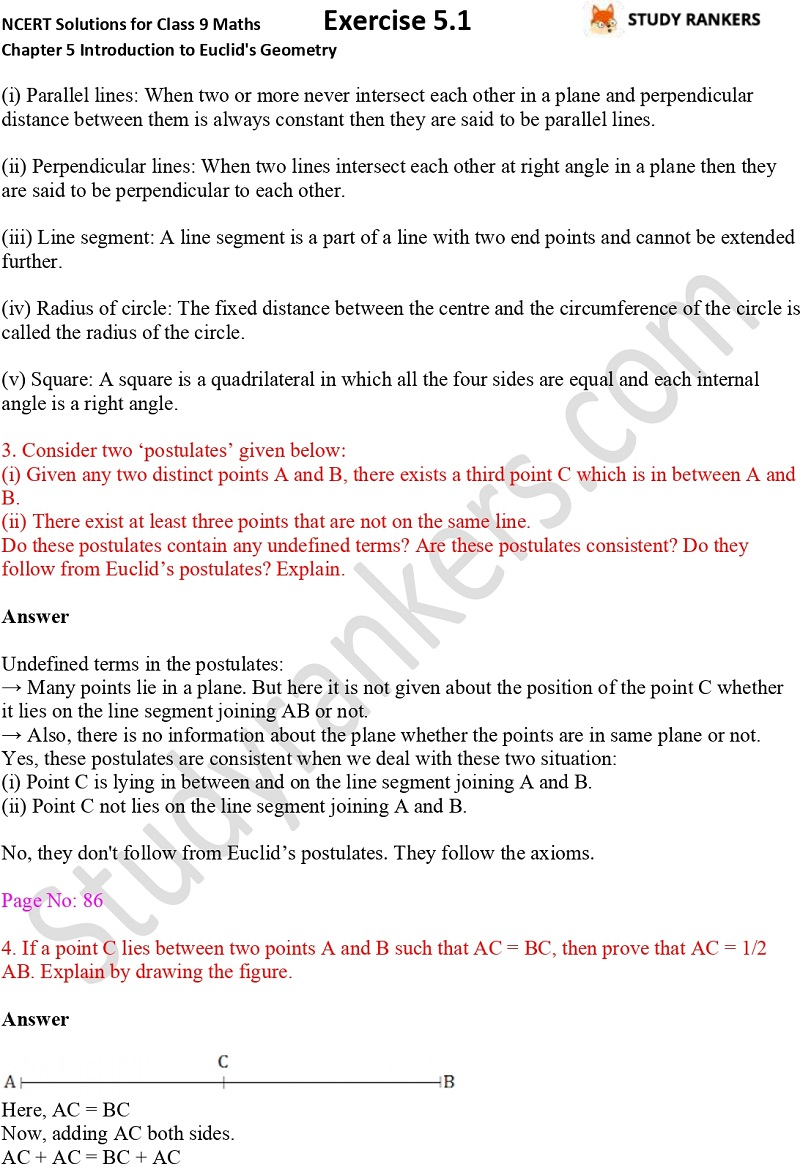## NCERT Solutions for Class 9 Maths Chapter 5 Introduction to Euclid's Geometry Exercise 5.1

Exercise 5.1 NCERT Solutions of Chapter 5 Introduction to Euclid's Geometry Class 9 Maths will be very useful in completing your task and improve your marks in the examinations. Practicing NCERT Solutions for Class 9 Maths will improve your problem solving skills thereby increasing your efficiency. These will be also helpful in revising the concepts of the chapter so you understand the important formulas.

Exercise 5.1 has seven questions in which you need to state whether given statements are true and false, draw specific figure, prove that every line segment has one and only one mid-point etc.